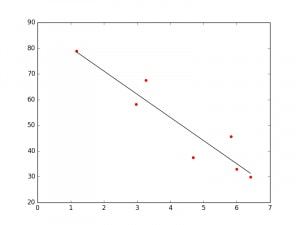In this tutorial, we will be building a basic linear regression that will indicate if there is a positive or negative relationship between two variables.

Interested in more advanced frameworks?  View our tutorial on Neural Networks in Python.

Understanding Linear Regression

A linear regression is a good tool for quick predictive analysis: for example, the price of a house depends on a myriad of factors, such as its size or its location. In order to see the relationship between these variables, we need to build a linear regression, which predicts the line of best fit between them and can help conclude whether or not these two factors have a positive or negative relationship. This can help us figure out if a factor such as the # of schools in the area might increase or decrease real estate values.

For our purposes today, we will be looking at something slightly more fun: the relationship between math test scores and the tissue concentration of LSD in a test-taker’s skin (Source: Wagner, Agahajanian, and Bing.1968. “Correlation of Performance Test Scores with Tissue Concentration of Lysergic Acid Diethylamide in Human Subjects.” Clinical Pharmacology and Therapeutics, Vol.9 pp 635-638.) This is a fairly simple data set that I downloaded from the University of Florida open data sets, a catalog of which can be found here.

This data will help illustrate how we can visually map the relationship between a dependent variable (in this case the test score) and the independent variable (LSD intake). A slightly modified version of the dataset itself can be found in the Github repo for this tutorial, alongside the Python code that is excerpted in this write-up.

Before we start, let us clarify the way a linear regression algorithm is put together: the formula for this equation is Y = a + bX, where X is the independent (explanatory) variable and Y is the dependent variable. If you’re still confused, think about the individual axes: the y variable always plots the “dependent” variable, so in our example, that would be the test score. The x variable is the independent variable, or what is sometimes called the “explanatory variable” — in this case, the amount of LSD currently in someone’s tissue sample.

We want to plot how much of the variation in the y variable (in this case test scores) can be explained by variation in the x variable (in this case the amount of LSD a test-taker has in their bloodstream).

For this example, we will be using the pandas and sci-kit learn libraries in Python in order to both calculate and visualize the linear regression in Python. Let’s write those up now:

import pandas as pd

import numpy as np

import matplotlib.pyplot as plt

from sklearn.linear_model import LinearRegression

Next up, we load in our data. The Github repo contains the file “lsd.csv” which has all of the data you need in order to plot the linear regression in Python. Let’s read those into our pandas data frame. The second line calls the “head()” function, which allows us to use the column names to direct the ways in which the fit will draw on the data.

In the last step of our data preparation, we will be extracting the data from the pandas data frame in a way that the “fit()” function will work and wherein we can implement a linear regression in Python.

X = data['Tissue Concentration'].values[:,np.newaxis]

y = data['Test Score'].values

model = LinearRegression()

model.fit(X, y)

The code above creates all of the necessary data that we can then plot using sci-kit learn. Let’s plot that now:

plt.scatter(X, y,color='r')

plt.plot(X, model.predict(X),color='k')

plt.show()

And voila! Below you should see your shiny new linear regression, which shows a negative correlation between LSD intake and math test scores (somewhat unsurprisingly).Linear regression in Python, Math Test Scores on the Y-Axis, Amount of LSD intake on the X-Axis.